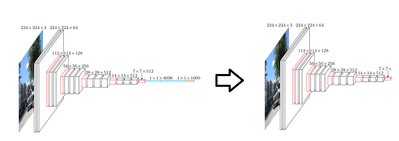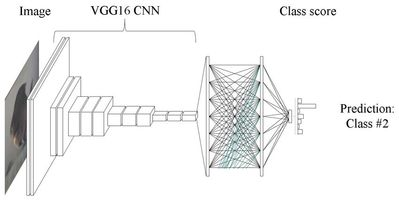Community Articles
Find and share helpful community-sourced technical articles.

Deep Learning in Medicine: Classifying Melanoma Part 2: Implementing with TensorFlow and Keras (Steve Kiaie)

Labels (2)

• Tensorflow

skiaieCloudera Employee

In part 1 of, we looked at how we could use deep learning to classify Melanoma, using transfer learning with a pre-trained VGG16 convolutional neural network. Here we take a look at how to implement this using TensorFlow and Keras.

First we import the packages we need:

import numpy as np
from keras.preprocessing.image import ImageDataGenerator
from keras.models import Sequential
from keras.layers import Dropout, Flatten, Dense
from keras import applications

These packages include, the network layers we will be using, the applications (which includes the pretrained VGG16 model), and some image pre-processing utilities.

Next, we “strip” our model of it’s top layers. This is as simple as one line of code in Keras.

base_model = applications.VGG16(include_top=False,
weights='imagenet')Next we take our images of skin, and we transform them using Keras image pre-processing utility:

datagen = ImageDataGenerator(
rotation_range=40,
width_shift_range=0.2,
height_shift_range=0.2,
rescale=1./255,
shear_range=0.2,
zoom_range=0.2,
horizontal_flip=True,
fill_mode='nearest')

This will provide us with extra variation in our training image data-set, and will help prevent overfitting in our model.

Next, we feed our processed images through our “stripped” VGG16 network, and generate a feature set.

generator = datagen.flow_from_directory(
validation_data_dir,
target_size=(img_width, img_height),
batch_size=batch_size,
class_mode=None,
shuffle=False)

bottleneck_features_validation = model.predict_generator(
generator, nb_validation_samples // batch_size)

Next we take build a fully connected classifier network.

top_model = Sequential()
top_model.compile(optimizer='rmsprop',
loss='binary_crossentropy', metrics=['accuracy'])

We train this classifier by feeding the feature set generated from our stripped VGG16 network through it. We’ll also save the weights of the trained classifier, once we’ve trained it.

top_model.fit(train_data, train_labels,
epochs=epochs,
batch_size=batch_size,
validation_data=(validation_data, validation_labels))
top_model.save_weights(top_model_weights_path)

Finally, we plug our classifier model back to our stripped VGG16 model, and we re-train the convolutional weights of the VGG16 network.model = Model(inputs=base_model.input, outputs=top_model(base_model.output))
model.compile(loss='binary_crossentropy',
optimizer=optimizers.SGD(lr=1e-4, momentum=0.9),
metrics=['accuracy'])
model.fit_generator(
train_generator,
steps_per_epoch=nb_train_samples // batch_size,
epochs=epochs,
validation_data=validation_generator,
validation_steps=nb_validation_samples // batch_size,
verbose=2)

In this case, our train_generator, and validation_generator’s are created in the same way we created the generator for our classifier.

You can see the full example, and build this for yourself on github, at:

https://github.com/hortonworks-sk/HDP-3.0-classifying-melanoma

1,183 Views
Don't have an account?
Announcements
What's New @ Cloudera
What's New @ Cloudera
What's New @ Cloudera
What's New @ Cloudera
Version history
Last update:
‎08-19-2019 01:31 PM
Updated by:Contributors
Top Kudoed Authors